PSABDZJZ - Grade 4 Mathematics Practice Test 2015

A pet store needs to put 23 birds into birdcages. Each birdcage can hold 4 birds.

What is the least number of cages the pet store needs to hold all the birds?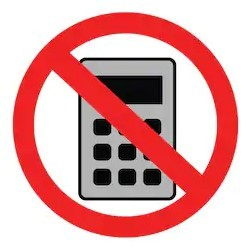Select one:

The table below shows the number of lunches sold each day for three days.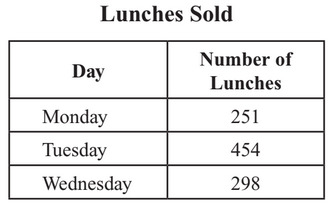Which of these has a value that is closest to the total number of lunches sold for the three days?Select one:

Dianne has the four number cards shown below.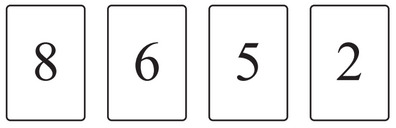Dianne used two of her cards to make a 2-digit number that is a multiple of 4.

What could be the number that Dianne made?Type your answer below as a number (example: 5, 3.1, 4 1/2, or 3/2):
Part A)

The fractionis shaded on the fraction model below.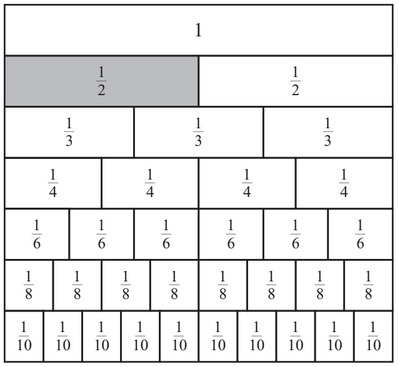Write two different fractions that are equivalent to.

Write one fraction here.Part B)

Write another fraction equivalent to.

Which model is a way to multiply 3 by 14?

Model A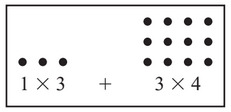Model B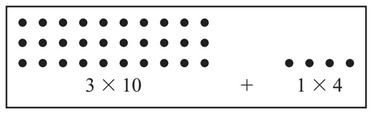Model C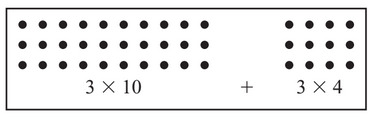Model D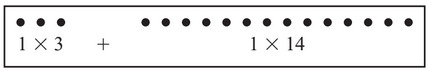Select one:

Which of these is true?Select one:

• It is a multiple of 2.
• Is is a factor of 18.
• It is a composite number.

Which of these numbers could be the mystery number?Select one:

Tameca scored 6 points in a basketball game. Leah scored 3 times as many points as Tameca in the basketball game.

Which equation shows the number of points Leah scored?Select one:

Ella collected 5 times as many bugs as Mari. Mari collected 15 bugs.

What was the total number of bugs Ella collected?Select one:
Part A)

For this question, you will draw three shapes on the gird.

On the grid, draw a parallelogram. Label your parallelogram A.

On a piece of paper, draw your grid and your parallelogram labeled A. Take a picture and upload it using the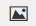button. If your cannot, write "Parallelogram is on paper."Part B)

On the grid, draw a triangle that has two sides that are perpendicular to each other.

• Put an X on each of the sides that are perpendicular.
• Explain how you know the sides you labeled X are perpendicular.

On a piece of paper, draw your grid and your triangle labeled B. Take a picture and upload it using thebutton. If your cannot, write "Triangle is on paper."Part C)

On the grid, draw a shape that has at least one pair of parallel sides, at least one pair of perpendicular sides, and only two right angles. Label your shape C.

On a piece of paper, draw your grid and your shape labeled C. Take a picture and upload it using thebutton. If your cannot, write "Shape is on paper."Part D)

What is the name of the mathematical shape your drew in part (c)?

A decimal number is missing from the number sentence below.

0.24 >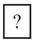Which of the following represents a decimal number that belongs in theto make the number sentence true?

A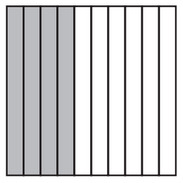B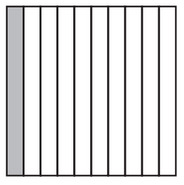C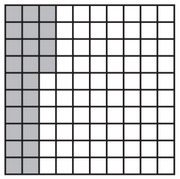D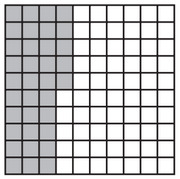Select one:

The picture below showspans of cookies that Reggie baked.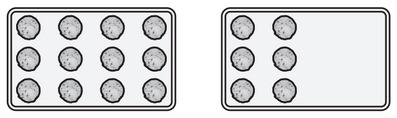Which of the following is another way of writing?Select one:

Mr. Jones put 162 books on the library shelves. There are 6 shelves. He put the same number of books on each shelf.

How many books did Mr. Jones put on each shelf?Select one:

Which equation is true?Select one:

Greta recorded the number of miles she walked each day last week on the line plot, as shown below.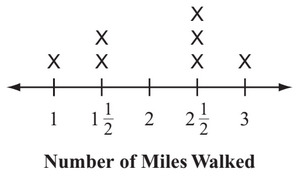How many miles in all did Greta walk last week?Select one:

What is the value of the 7 in 472,582?Select one:

Draw an angle that measures 180°.

On a piece of paper, draw a 180° angle. Take a picture and upload it using thebutton. If you cannot, write "Angle is on paper."Which of the following has the greatest number of lines of symmetry?

Shape A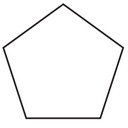Shape B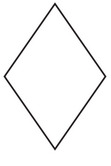Shape C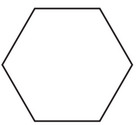Shape D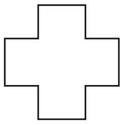Select one:

Lisa shadedof the square shown below.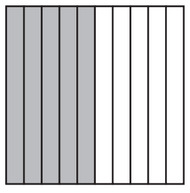Which number shows the part of the square that Lisa shaded?Select one:

Connor wrote the equation shown below.

6 x 8 = n

Which statement about Connor's equation is true?Select one:
Part A)

The length and width of a field are shown in the diagram below.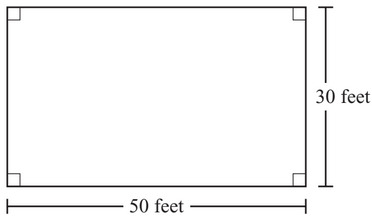What is the perimeter, in feet, of the field? Show or explain how you got your answer.